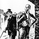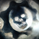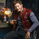2117 views
2117
This indicator was designed to remove trend from price and make it easier to identify cycles.
Although this indicator has similarities to MACD . It is better used to identify the cycle of High and Lows based on the Statistical Data (Default is set to 25).

**** DO NOT USE THIS AS A MOMENTUM INDICATOR ****

As a word of caution the read this - http://bit.ly/1Arjoqp. This is a rip off, if you choose to pay a penny.
```// Created by UCSgears
// Version 1 - Setting for the Power cycle is not determined yet. This is the concept.
// Larry Gaines version of Cycle uses Linear Regression

study(title="UCS_Cycle_V1", shorttitle="UCS-Cycle", overlay=false)

// Classic Cycle Indicator uses SMA
UseLinearRegression = input(true)
PlotSignal = input(false)

// The Source can be Based on OHLC4 or HL2
src = (ohlc4)
len = input(25, title="Cycle Length")
smooth = input (8, title = "Signal")
basis = UseLinearRegression ? linreg(src, len, 0) : sma(src,len)

cycle = close - basis
cyclesig = PlotSignal ? ema(cycle,smooth) : 0

plot_color1 = cycle > 0 ? green : red

plot(cycle, color = plot_color1, title = "Linear Regression Curve", style = columns, linewidth = 4)
plot(cyclesig, color = gray, title = "Signal")

//END
// Version 2 will have all the settings for the 3 Power Cycle Bands with Options to Plot them all.```Would be possible to colour the bars green/red depending on if they are above or below the zero line?TheYangGuizi
add the below code after the plot statements

barcolor (plot_color1)Hi guys...when will Version 2 come out? Great script!